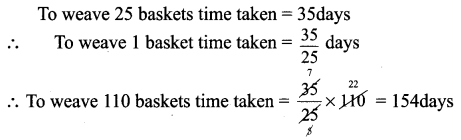Students can Download Maths Chapter 4 Direct and Inverse Proportion Additional Questions and Answers, Notes Pdf, Samacheer Kalvi 7th Maths Book Solutions Guide Pdf helps you to revise the complete Tamilnadu State Board New Syllabus and score more marks in your examinations.

## Tamilnadu Samacheer Kalvi 7th Maths Solutions Term 1 Chapter 4 Direct and Inverse Proportion Additional Questions

Exercise 4.1

Question 1.
The amount of extension in an elastic spring varies directly as the weight hung on it. If a weight of 150 gm produces an extension of 2.9 cm, then what weight would produce an extension of 17.4 cm?
Solution:
To produce 2.9 cm extension weight needed = 150 gm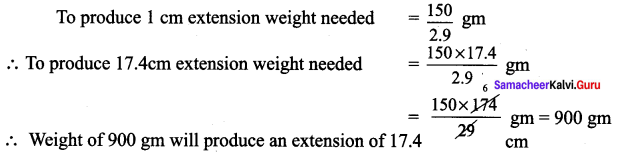Question 2.
Reeta types 540 words during half on hour. How many words would she type in 12 minutes?
Solution:
In $$\frac{1}{2}$$ an hour number of words typed = 540
i.e., In 30 min No. of words typed = 540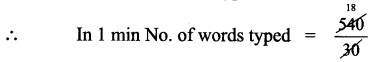= 18
In 12 minutes number of words typed = 18 × 12
= 216
216 words can be typed in 12 min

Question 3.
A call taxi charges ₹ 130 for 100 km. How much would one travel for ₹ 390?
Solution: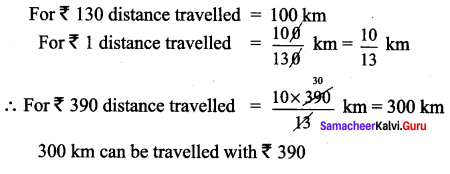Exercise 4.2

Question 1.
In the following table find out x and y vary directly or inversely?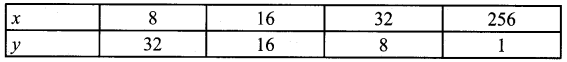Solution:
From the table itself we observe that as x increases y decreases.
∴ x and y are inversely proportional
∴ xy = 8 × 32 = 16 × 16 = 32 × 8 = 256 × 1 = 256

Question 2.
If x and y vary inversely as each other and x = 10 when y = 6. Find y when x = 15.
Solution:
Since x and y vary inversely as each other
xy = constant
10 × 6 = 15 xy
60 = 15y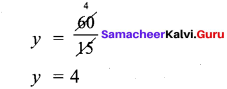Question 3.
If x and y vary inversely and if y = 35 find x when constant of variation is 7.
Solution:
Given x andy are inversely proportional
xy = constant
when y = 35 and constant = 7 ; x × 35 = 7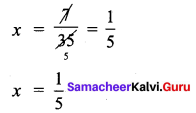Exercise 4.3

Question 1.
Sumathi sweeps 600 m long road in 2$$\frac{1}{2}$$ hrs. Ramani sweeps $$\frac{2}{3}$$ rd of same road in 1$$\frac{1}{2}$$ hrs. Who sweeps more speedily?
Solution: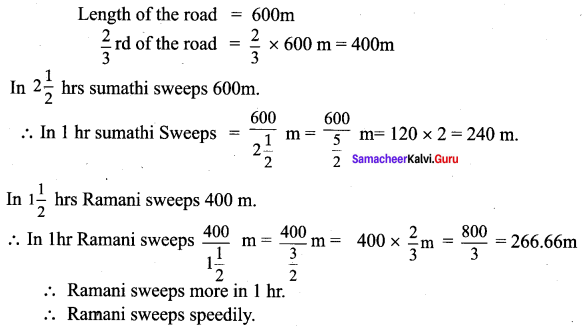Question 2.
Suma weaves 25 baskets in 35 days. In how many days will she weave 110 baskets?
Solution: